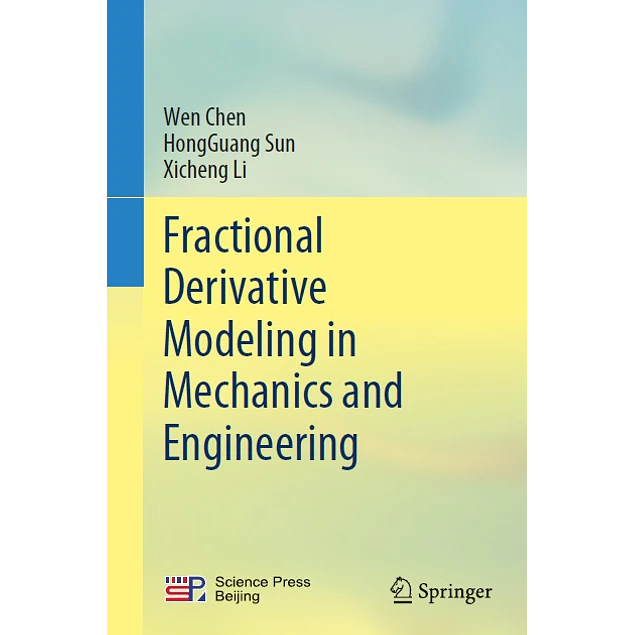### Order this book through WhatsApp. Click now to chat.# Fractional Derivative Modeling in Mechanics and Engineering

1st ed. 2022 Edition

by Wen Chen (Author), HongGuang Sun (Author), Xicheng Li (Author)

This textbook highlights the theory of fractional calculus and its wide applications in mechanics and engineering. It describes in details the research findings in using fractional calculus methods for modeling and numerical simulation of complex mechanical behavior. It covers the mathematical basis of fractional calculus, the relationship between fractal and fractional calculus, unconventional statistics and anomalous diffusion, typical applications of fractional calculus, and the numerical solution of the fractional differential equation. It also includes latest findings, such as variable order derivative, distributed order derivative and its applications. Different from other textbooks in this subject, the book avoids lengthy mathematical demonstrations, and presents the theories in close connection to the applications in an easily readable manner. This textbook is intended for students, researchers and professionals in applied physics, engineering mechanics, and applied mathematics. It is also of high reference value for those in environmental mechanics, geotechnical mechanics, biomechanics, and rheology.

Year:
2022
Pages:
381
Language:
English
Format:
PDF
Size:
5 MB
Publisher:
Springer
ISBN-10:
981168801X
ISBN-13:
978-9811688010, 9789811688010
ASIN:
B09TJ19CLJ
Tag: